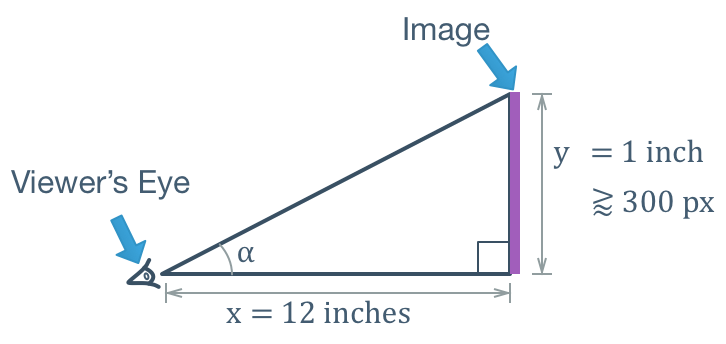# What is the Right Resolution for Life-Sized Prints?

I came from a background of small print projects. Now I’m tasked to find photography art for five doors, each 12 x 14 inches. When it comes to finding images, I don’t know where to start. What would be the best size? Best resolution?For a start, there’s plenty of royalty-free images that you can find from Pexels, Pixabay, or Unsplash. Most photographs from these sources would have good lighting as well. Hence you just need to pick the ones which has enough pixels for your given print project.

Since our dear reader was looking for images to place on doors, I’m going to assume that people would view these images no closer than at an arm’s length. This would be about two feet or 24 inches.

Why does the distance matter? Image resolution is not just how many dots to put in a given inch (the dot per inch or pixel per inch metric), but also how far people would view them. The further the viewing distance, the less pixel density would be required such that people who view them doesn’t perceive the image as blurry, blocky, or otherwise be able to identify the individual pixels (dots) in the image.

What you need is a function that estimates the lower bound pixel density given an expected viewing distance. In other words, a formula that converts a distance in inches into a minimum dots-per-inch density value.

```q = f(x)
```

Where:

• x  — the expected distance between the viewer and the image, in inches.
• q  — the minimum image resolution, in dots-per-inch or pixels-per-inch.

To derive the formula, first let’s draw a schematic of the problem. The image below shows a human eye (representing the viewer) that is looking at an image of height y at distance x. Now the angle that the eye makes between the bottom of the image and its top is . Let’s keep things simple and two dimensional for now, because making calculations for the width of the image would be exactly the same way, only on a different axis.We know that magazines are usually printed at 300 dpi (dots per inch). This resolution is effective when a person with normal eyesight is viewing it on a standard reading distance, which is one foot (12 inches). This is probably also the reason why the first retina-display iPhone debuted at that approximate pixel density, which is 326 ppi (pixels per inch). Similarly a retina-display MacBook Pro has only 220 ppi but looks just as good because of the longer viewing distance. Of course, some people has better eyesights and can appreciate higher resolution images whereas some others can’t tell the difference between iPhone 4 and iPhone 3G (before retina display) screens. But just take the middle ground for simplicity’s sake.

Now let’s plug in those standard numbers into our model. Let’s take an image of 1 inch high which is viewed at a distance of 12 inches. The image contains 300 pixels, which is the minimum required to “look good”.Then we need to find angle that the eye makes between looking at the bottom and the top edge of the image. This would be useful to calculate the pixel per degree value later on. Using knowledge from high-school trigonometry, we get…

```tan(⍺)  = y/x
tan(⍺)  = 1 inch / 12 inch

⍺ = arctan(1/12)
⍺ ≅ 4.76°
```

We’ll define p as the pixel-per-degree ratio as follows:

```p = q / ⍺
```

Where

• p — the pixel-per-degree ratio
• q — the number of pixels (or printed dots).
•  — the angle which contains those pixels.

Our 1-inch image has the bare minimum of q=300 pixels (dots), making it a 300dpi image. Let’s plug those numbers in.

```p = q / ⍺
p = 300 pixels / 4.76°
p ≅ 62.98 pixels/degree
```

That means that for every degree of eye movement there need to be at least `p ≅ 62.98` pixels between them so that the eye can’t differentiate the individual pixels.

Now we want an arbitrary distance x to calculate how many pixels q that our 1-inch image can look acceptable. We solve the two equations above for the value of q.

```p = q / ⍺
q = p * ⍺

⍺ = arctan(y/x)
q = p * arctan(y/x)
```

We already know the following values derived from our standard model of 1 inch image at a resolution of 300 pixels per inch viewed at 12 inch distance.

```y = 1 inch
p = 62.98 pixels / degree
```

Plug those numbers in and you get the formula to calculate the minimum resolution given an expected viewing distance. This is the formula that we’ve been looking for, the function to convert a viewing distance to a minimum pixel density value.

```q = 62.98 * arctan(1 / x)
```

Where

• q — the minimum resolution in pixels or dots per inch.
• x — the expected distance between the image and the viewer.

Then let’s go back to our dear reader’s initial problem. He/she would need to find images to fill 12 * 14 inch surfaces on doors. We’re assuming people won’t observe these doors closer than an arm’s length (or otherwise risking getting hit by the door if someone else is opening it from the other side), thus the viewing distance is about 24 inches (two feet).

```q = 62.98 * arctan(1/24) ≅ 150 pixels per inch.
```

Given these we can calculate the minimum pixel dimensions of the images for the doors:

```width  = 12 inches * 150 pixels per inch = 1800 pixels
height = 14 inches * 150 pixels per inch = 2100 pixels
```

Fortunately most images in Pexels, Pixabay, or Unsplash has more pixels than the minimum dimensions above and thus would look good to be printed on doors.